# Measurement of Capacitance By Transformer Ratio Bridge

0
279

#### A circuit for measurement of capacitance By Transformer Ratio Bridge is given in the below figure.An unknown capacitance C is measured in comparison with a standard capacitance C, which is assumed to be perfect.Resistance R represents the loss of the capacitor.Since for balance, the magnitude and phase of the currents passing through the detector should be the same, a variable standard resistance is connected in parallel with the standard capacitor.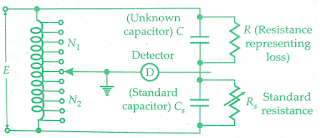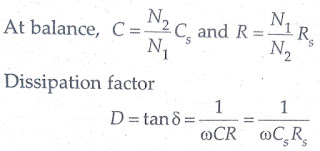## Measurement of Phase Angle:

#### A circuit for measurement of small phase angles by ratio transformers bridge is shown in the below figure.An RC circuit is used where the capacitance is variable in order to get phase shift.The value of resistance should be large in order that there are no loading effects on the ratio transformer.The capacitance is changed till the detector indicates null.#### The magnitude of in-phase component is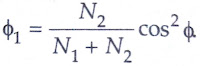## Transformer Double Ratio Bridges:

#### An alternate way of indication of balance is that the current flowing through the unknown impedance is equal and opposite to that through the standard or known impedance.The detector indicates this condition of equality. The arrangement of Transformer Double Ratio Bridges is shown in the below figure.It consists of an ideal voltage transformer having a secondary winding of N1 turns.The secondary winding is tapped at N2 turns.The resulting voltages E1 and E2 are applied across the unknown impedance, Z, and standard impedance, Zs, respectively.#### At balance, the two mmfs,  n1I1 = n2I2#### If the unknown impedance is a pure resistance R, a standard resistance Rs is required for obtaining balance#### If the unknown impedance is a pure capacitance C, a standard capacitor Cs is required for balance,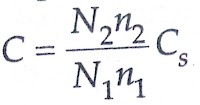#### Unknown conductance,#### where Gs is the standard conductance.

In practice, the capacitors are not perfect, the losses being represented by a conductance in parallel with the capacitance.Therefore, the balance has to be obtained both for resistance as well as for capacitance.In other words, mmf equality of both magnitude as well as for phase has to be obtained in order to get balance.A circuit used for measurement of the imperfect capacitor in terms of the standard capacitor and standard conductance is shown in the below figure.

#### Under balance,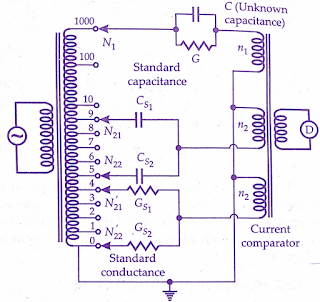## Measurement of Inductance:

#### In order to obtain mmf balance due to unknown inductance and the standard capacitance, it is necessary to reverse the comparator winding connected to the standard capacitor.This condition of bridge operation is normally indicated by the addition of a negative sign to the display of capacitance Cm.Suppose an inductance Lp in parallel with a resistance Rp represents the actual inductor having an inductance L in series with a resistance R.## Measurement of Components in ‘SITU’:

#### One of the greatest advantages of double ratio transformer bridges is their capability to measure the values of the components while they remain connected in the circuit.The below figure shows a circuit for measurement of an unknown impedance Z.#### If the ratio transformers are considered ideal the values of impedances Ze and Zi can be assumed to be zero and the voltage applied to the unknown impedance Z is not affected by current flowing in impedance Z1.Also, all the current flowing through Z would flow into winding as :#### The value of unknown approximately given by :#### In practice impedances, Ze and Zi have small finite values and corrections must be applied for this.In order that corrections are applied Ze and Zi must be determined.

READ HERE  Star Delta Starter? Working principle,Theory, Circuit Diagram